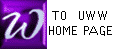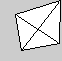a Simplex
SIMPLEX METHOD
APPLIED TO MINIMIZING
and NON-STANDARD PROBLEMS

INDEX
OF
TERMS
 PIVOT Constraint Objective Function Simplex Tableau Standard Maximizing Problem Hopping phase I Objective row Ratios (Quotients) Identity Sub-Matrix (ISM) Indicator Z-column basic solution Final Tableau pivoting , pivot operation

Definitions
 A linear programming problem (or linear program) is a set of (linear) inequalities (with a solution set S) and a (linear) function (often cost or profit) whose value (within S) is to be maximized or minimized.
A STANDARD MAXIMIZING PROBLEM is a linear programming problem
which satisfies all of the following 4 CONDITIONS (Rolf, pg.263) :
 [C1] The objective function is to be maximized. [C2] All variables are non-negative. [C3] All inequalities are of the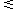type. [C4] All right hand constants are non-negative.
 A NON-STANDARD PROBLEM is simply a problem which is not standard, and hence fails to satisfy at least one of [C1] through [C4] above. In our text, [C2] is always true, which simplifies discussions.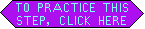Reference : An example of how to apply the following procedure to a non-standard problem is available, with abundant comments and cross-references.

Reference : Many EXERCIZES are available for each step of this method.

 Step NS-1
 Convert your problem into one satisfying conditions C1,C2, and C3 described above. How to do this is explained in Rolf 8th edition (pg 318-319).

 Step NS-2
Convert all constraints to equations with slack variables, and then write the problem as a tableau with some negative right sides, with or without a Z-COLUMN.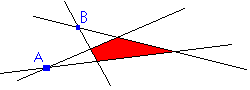Strategy
 The basic solution for a tableau with some negative right sides is a point like A or B in the figure above : it will not be a corner of the RED shaded solution set, but rather will be an intersection of extended boundaries of that set. Our first task will be to locate a corner point of the actual solution set : this task might be called PHASE I and is described here : it differs from the procedure for a standard problem only in STEPS NS-1 and NS-3. After locating some corner of the solution set (shaded above), we must set out to locate the BEST corner : this second and final stage of the solution is called PHASE II, and is identical to the procedure for a standard problem.
Note
 PHASE I, as described in Rolf 8th edition (Step 5, Pg 329), allows the freedom to choose any number as pivot. On tests, you must follow the more restrictive PHASE I procedure given below, not the unrestricted procedure in Rolf.

FINDING THE PIVOT (steps NS-3 through NS-6)

 Step NS-3
Locate the row containing the most negative right-hand number. This row will be called the INDICATOR ROW. If no right-hand number is negative, then your tableau is STANDARD.Step NS-4
INDICTORS will consist of all numbers in the row found in NS-3, except the right-most number; the PIVOT COLUMN will contain the most negative of these indicators. If no indicator is negative, then there is no pivot column, and the problem is unsolvable.Step NS-5
Form RATIOS or QUOTIENTS for all (non-objective) rows : for each row, divide the right-most number by the number in the pivot column.Step NS-6
The PIVOT will be in the ROW with smallest non-negative ratio. Note that 0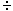(+1) and 0(-1) are both numerically zero, but in calculating RATIOS, consider 0(+1) as positive (OK), and 0(-1) as negative (not OK).Step NS-7
Perform a pivot transformation on the above pivot (Rolf, Pg 98). Check out the PIVOT ENGINE to speed pactice.Step NS-8
If all right-most (non-objective row) entries are non-negative, then phase I is ended. PHASE II now consists of applying steps 3-9 of the standard maximizing procedure to the new tableau obtained in step NS-7 above. Note: PHASE II is described as step #7 on Page 329 of our text, Rolf.

 Step NS-9
Otherwise, if some right-most (non-objective row) entry is negative, apply steps NS-3 through NS-9 to the new tableau obtained in NS-7.